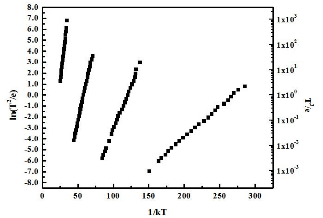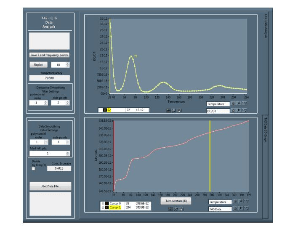Thermal admittance spectroscopy (TAS) uses electrical characterization to measure the capacitance and conductance as a function of frequency and temperature. TAS is a steady state measurement, in contrast to the non-equilibrium measurements made in DLTS. Also, where DLTS is used for doped semiconductors, TAS may also be used for low-doped or high resistivity material.

The concept behind TAS is to measure the capacitance and conductance (impedance) over a range of frequencies and temperature. At a high-test signal frequency, a deep trap is not able to respond and contribute to the capacitance and conductance if the emission rate is slower than the test frequency. As the temperature increases, the emission rate of a defect increases, and the trap can contribute to the measured.

At a threshold in frequency or temperature, the deep level signal appears as a step in the capacitance, or a peak in the conductance. The temperature of the inflection point of each plot of capacitance versus temperature at a fixed frequency is used along with the frequency, as one point in an Arrhenius plot for each frequency. The energy of the defects can be obtained from plotting pairs of frequency and temperature points in an Arrhenius plot of emission rate (frequency of measurement) versus 1/kT.

TAS data acquisition is performed easily, and the analysis program facilitates efficient extraction of defect characteristics with the Semetrol TAS system. TAS is a method that is complementary to the DLTS method. TAS is applicable to low-doped semiconductors, where DLTS is generally applicable to moderately doped material.

Recent improvements to the programs include acquiring impedance and phase angle in the data acquisition, rather than just focusing on one component of impedance. Analysis is free to use any of the components, real or imaginary, and in either series or parallel mode.Energy measurements are very accurate with TAS, generated from emission rate measurements over several orders of magnitude.Analysis consists of finding the steps in capacitance, or peaks in conductance from a plot of the capacitance or conductance measured over temperature, at each frequency. The pairs of coordinates of frequency and temperature are then used to generate the Arrhenius plot and measure the energy of the traps.